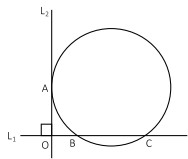# Free GMAT Test Questions

Question 1 of 1
ID: GMAT-PS-9
Section: Quantitative Reasoning - Problem Solving
Topics: Geometry; Circle
Difficulty level: Challenging

(Practice Mode: Single selected Question » Back to Overview)

In the figure below, the straight lines L${}_{1}$ and L${}_{2}$ intersect each other at right angles. The circle touches the line L${}_{2}$ at A and intersects the line L${}_{1}$ at B and C. If OA = 4 and BC = 6, what is the radius of the circle?A2
B3
C4
D5
E6